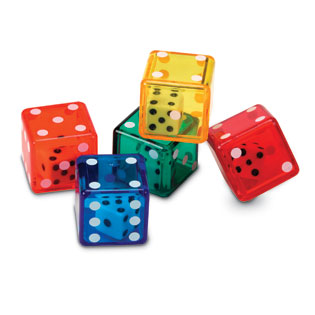The first BorelCantelli lemma is one of rare elementary providers of almost sure convergence in probability theory. It states that if ${(A_n)}$ is a sequence of events in a probability space ${(\Omega,\mathcal{F},\mathbb{P})}$ such that ${\sum_n\mathbb{P}(A_n)<\infty}$ then ${\mathbb{P}(\varlimsup_nA_n)=0}$. The classical proof consists in noticing that ${B_n=\cup_{m\geq n}A_m}$ is non increasing in ${n}$, and thus, since ${\sum_n\mathbb{P}(A_n)<\infty}$,

$\mathbb{P}(\varlimsup A_n) =\mathbb{P}(\cap_nB_n) =\lim_n\mathbb{P}(B_n) \leq \lim_{n}\sum_{m\geq n}\mathbb{P}(A_m)=0.$

There is another proof of this lemma that I prefer, and which goes as follows: by the Fubini-Tonelli theorem or by the monotone convergence theorem,

$\mathbb{E}(\sum_n\mathbf{1}_{A_n}) =\sum_n\mathbb{E}(\mathbf{1}_{A_n}) =\sum_n\mathbb{P}(A_n) <\infty$

therefore ${\mathbb{P}(\sum_n\mathbf{1}_{A_n}=\infty)=0}$. Now ${\{\sum_n\mathbf{1}_{A_n}=\infty\}=\varlimsup A_n}$. I like very much this proof because it concentrates useful ingredients for students:

• we count by summing indicators of events and ${\{\sum_n\mathbf{1}_{A_n}\}=\varlimsup A_n}$
• ${\mathbb{E}(\mathbf{1}_A)=\mathbb{P}(A)}$
• Fubini-Tonelli allows to swap ${\mathbb{E}}$ and ${\sum}$
• if ${X\geq0}$ and ${\mathbb{E}(X)<\infty}$ then ${\mathbb{P}(X=\infty)=0}$

A very similar argument may be used to obtain quickly a strong law of large numbers. Namely, if ${(X_n)}$ are independent centered random variables bounded in ${\mathbf{L}^4}$ (i.e. ${\sup_n\mathbb{E}(|X_n|^4)<\infty}$) and if we set ${S_n:=\frac{1}{n}(X_1+\cdots+X_n)}$, then by expanding and using the assumptions we get ${\mathbb{E}(S_n^4)=\mathcal{O}(n^{-2})}$, which gives

$\mathbb{E}(\sum_nS_n^4) =\sum_n\mathbb{E}(S_n^4) <\infty$

and thus ${\mathbb{P}(S_n\rightarrow0)\geq\mathbb{P}(\sum_nS_n^4<\infty)=1}$. To me, this is a bit more elegant than using the first Borel-Cantelli lemma and the Markov inequality.

Last Updated on

This site uses Akismet to reduce spam. Learn how your comment data is processed.

Syntax · Style · .